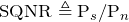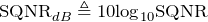# Signal-to-Quantization Noise Ratio (SQNR)

November 6, 2019

Signal power and quantization noise should be discussed in unison. Most importantly, the signal power should be sufficiently more than the power of the quantization noise. The signal-to-quantization noise ratio (SNQR) is used to equate this ratio.

### SQNR

As discussed in the previous lesson, quantization noise is added when quantization is used to digitize a signal. Some level of error is introduced with this method of analog-to-digital conversion. The SQNR is used as a quick error metric in regard to quantization noise.

#### Equation

Let Ps be the signal power and Pn be the quantization noise. The SQNR and its decibel version, SQNRdB, are defined as:

(1)(2)#### Increasing the Signal-to-Quantization Noise Ratio

A higher SQNR value indicates a lower value of quantization noise. The SQNR value can be increased in several ways, two of which are discussed in the following lessons.